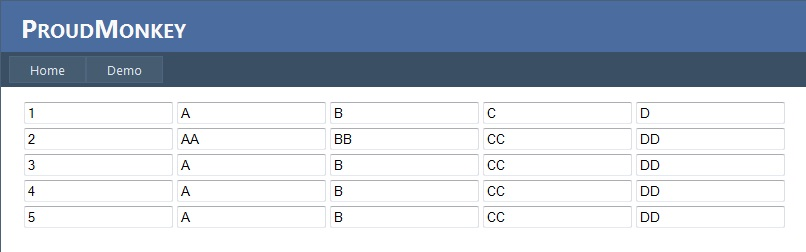Geeks With Blogs
Vinz' Blog (ProudMonkey) "Code, Beer and Music ~ my way of being a programmer"

Few months ago I wrote an example about Dynamically Adding TextBox Control to ASPNET Table. As a recap that example demonstrate how to generate rows of TextBoxes in a Table control based on the number of rows and number of columns provided and as well as print the values entered by the user using the Request.Forms collections.. Recently,one of the user is asking  if how are we going to accomplish the same thing by generating the TextBox in the Table based on the data from the database. So in this post I'm going to demonstrate an example on how to do it.

Okay to get started lets go a head and set up our WebForm.  I have used master page in this example so the mark up would look something like this:

`   1:  <asp:Content ID="Content1" ContentPlaceHolderID="HeadContent" runat="server">`
`   2:  </asp:Content>`
`   3:  <asp:Content ID="Content2" ContentPlaceHolderID="MainContent" runat="server">`
`   4:      <asp:PlaceHolder ID="PlaceHolder1" runat="server"></asp:PlaceHolder>`
`   5:  </asp:Content>`

As you can see there's nothing fancy in the mark up above. It just contain a PlaceHolder control in which we will add the dynamic table on it. Now lets flip to code behind page.

Here are the full code blocks below:

`   1:          private DataTable FillData() {`
`   2:   `
`   3:              DataTable dt = new DataTable();`
`   4:              DataRow dr = null;`
`   5:   `
`   6:              //Create DataTable columns`
`   7:              dt.Columns.Add(new DataColumn("RowNumber", typeof(string)));`
`   8:              dt.Columns.Add(new DataColumn("Col1", typeof(string)));`
`   9:              dt.Columns.Add(new DataColumn("Col2", typeof(string)));`
`  10:              dt.Columns.Add(new DataColumn("Col3", typeof(string)));`
`  11:              dt.Columns.Add(new DataColumn("Col4", typeof(string)));`
`  12:   `
`  13:              //Create Row for each columns`
`  14:              dr = dt.NewRow();`
`  15:              dr["RowNumber"] = 1;`
`  16:              dr["Col1"] = "A";`
`  17:              dr["Col2"] = "B";`
`  18:              dr["Col3"] = "C";`
`  19:              dr["Col4"] = "D";`
`  20:              dt.Rows.Add(dr);`
`  21:   `
`  22:              dr = dt.NewRow();`
`  23:              dr["RowNumber"] = 2;`
`  24:              dr["Col1"] = "AA";`
`  25:              dr["Col2"] = "BB";`
`  26:              dr["Col3"] = "CC";`
`  27:              dr["Col4"] = "DD";`
`  28:              dt.Rows.Add(dr);`
`  29:   `
`  30:              dr = dt.NewRow();`
`  31:              dr["RowNumber"] = 3;`
`  32:              dr["Col1"] = "A";`
`  33:              dr["Col2"] = "B";`
`  34:              dr["Col3"] = "CC";`
`  35:              dr["Col4"] = "DD";`
`  36:              dt.Rows.Add(dr);`
`  37:   `
`  38:              dr = dt.NewRow();`
`  39:              dr["RowNumber"] = 4;`
`  40:              dr["Col1"] = "A";`
`  41:              dr["Col2"] = "B";`
`  42:              dr["Col3"] = "CC";`
`  43:              dr["Col4"] = "DD";`
`  44:              dt.Rows.Add(dr);`
`  45:   `
`  46:              dr = dt.NewRow();`
`  47:              dr["RowNumber"] = 5;`
`  48:              dr["Col1"] = "A";`
`  49:              dr["Col2"] = "B";`
`  50:              dr["Col3"] = "CC";`
`  51:              dr["Col4"] = "DD";`
`  52:              dt.Rows.Add(dr);`
`  53:   `
`  54:              return dt;`
`  55:          }`
`  56:   `
`  57:          private void GenerateTable(DataTable dtSource) {`
`  58:              int rowsCount = dtSource.Rows.Count;`
`  59:              int colsCount = dtSource.Columns.Count;`
`  60:   `
`  61:              //Creat the Table and Add it to the Page`
`  62:              Table table = new Table();`
`  63:              table.ID = "Table1";`
`  64:   `
`  65:              // Add the dynamic table to the PlaceHolder control`
`  66:              PlaceHolder1.Controls.Add(table);`
`  67:   `
`  68:              // Now iterate through the datatable and add the controls`
`  69:              for (int rowIndex = 0; rowIndex < rowsCount; rowIndex++) {`
`  70:   `
`  71:                  TableRow row = new TableRow();`
`  72:   `
`  73:                  for (int colIndex = 0; colIndex < colsCount; colIndex++) {`
`  74:                      TableCell cell = new TableCell();`
`  75:                      TextBox tb = new TextBox();`
`  76:                      // Set a unique ID for each TextBox added`
`  77:                      tb.ID = "TextBoxRow_" + rowIndex + "Col_" + colIndex;`
`  78:                      // Assign value for each TextBox added`
`  79:                      tb.Text = dtSource.Rows[rowIndex][colIndex].ToString();`
`  80:                      // Add the control to the TableCell`
`  81:                      cell.Controls.Add(tb);`
`  82:                      // Add the TableCell to the TableRow`
`  83:                      row.Cells.Add(cell);`
`  84:                  }`
`  85:                  // After the columns iteration, add the TableRow to the Table`
`  86:                  table.Rows.Add(row);`
`  87:              }`
`  88:          }`
`  89:          protected void Page_Load(object sender, EventArgs e) {`
`  90:              if (!IsPostBack) {`
`  91:                  GenerateTable(FillData());`
`  92:              }`
`  93:          }`

The code above have two main Methods which are the FillData() and GenerateTable(). The FillData() method is a method that returns a DataTable. If you notice I just fill the DataTable with a dummy data just for the purpose of this demo. In case you want to grab the data from the Database then you can replace the implementation of the FillData() method. You can use DataTable and  SqlDataAdapter. Assign a select command to your SqlDataAdapter and then callSqlDataAdapter .Fill method. This would populate the desired data into yourDataTable . Here's a quick example below for your reference:

`   1:         private DataTable FillData() {`
`   2:              DataTable dt = new DataTable();`
`   3:              using (SqlConnection connection = new SqlConnection("Your Connection String")) {`
`   4:                  try {`
`   5:                      connection.Open();`
`   6:                      string spName = "YOURStoredProcudureName";`
`   7:                      SqlCommand sqlCmd = new SqlCommand(spName, connection);`
`   8:                      SqlDataAdapter sqlDa = new SqlDataAdapter(sqlCmd);`
`   9:                      sqlCmd.CommandType = CommandType.StoredProcedure;`
`  10:   `
`  11:                      sqlDa.Fill(dt);`
`  12:                      if (dt.Rows.Count > 0) {`
`  13:                          return dt;`
`  14:                      }`
`  15:                  }`
`  16:                  finally {`
`  17:                      connection.Close();`
`  18:                  }`
`  19:                  return dt;`
`  20:              }`
`  21:          }`

That simple. Now, the GenerateTable() method handles the generation of dynamic texboxes in the Table control. This method will generate rows of TextBoxes based on the row count and column count of the DataTable source. I have included some comments on that method to help you undertand the code. Here's the output below when viewed in the browser:That's it! I hope someone find this post useful!